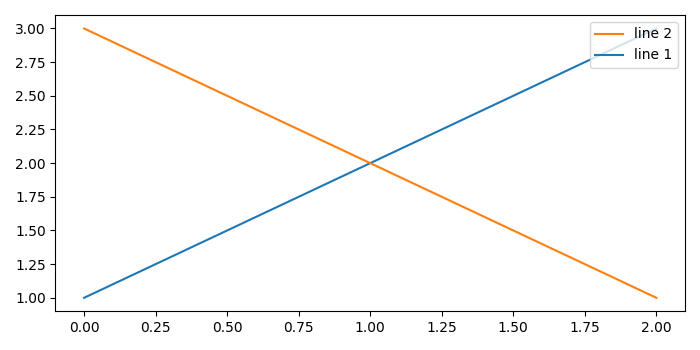# How to create a draggable legend in Matplotlib?

To create a draggable legend in matplotlib, we can take the following steps −

• Create two lines, line1 and line2, using plot() method.

• Place the legend for plot line1 and line2 with ordered lables at location 1, using legend() method.

• To create a draggable legend, use set_draggable() method, where state=True. If state=False, then we can't drag the legend.

• To display the figure, use show() method.

## Example

from matplotlib import pyplot as plt
plt.rcParams["figure.figsize"] = [7.00, 3.50]
plt.rcParams["figure.autolayout"] = True
line1, = plt.plot([1, 2, 3])
line2, = plt.plot([3, 2, 1])
leg = plt.legend([line2, line1], ["line 2", "line 1"], loc=1)
leg.set_draggable(state=True)
plt.show()

## OutputOn the output window, you can drag the legend around with the mouse.